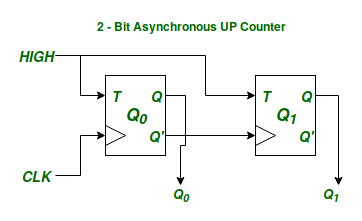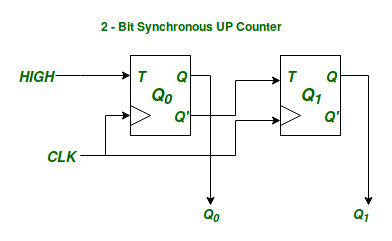Related Articles
Difference between Synchronous and Asynchronous Sequential Circuits
• Last Updated : 27 Dec, 2018

Sequential Circuits are those which have the notion of an internal state. This notion of Internal State is necessary because in sequential circuits, the output of the circuit is function of both the present input as well as the past inputs. The Internal State of a sequential circuit is nothing but the reflection of the past inputs to the circuit. Now the Internal State of a Sequential Circuit is represented by a number of State Variables. Each state variable can be in 1 of 2 possible states. This is because State Variables are physically implemented with the help of Flip-Flops, and each Flip-Flop can only represent 2 possible states. Therefore, if we have ‘N’ Flip-Flops, we can represent a maximum of 2N states.

`Max. No. of States with 'N' Flip-Flops =`

This means that a Sequential Circuit having ‘N’ Flip-Flops can be in at mostInternal States.

Now let’s illustrate the difference between that of Synchronous and Asynchronous Sequential Circuits with the example of a Synchronous and Asynchronous 2-bit binary UP Counter using T-Flip-Flops.Figure – 2-bit Binary Asynchronous UP CounterFigure – 2-bit Binary Synchronous UP Counter

In both the above circuitsare the State Variables denoting the Internal State of each of the above circuits. Since there are 2 state variable the above sequential circuits can be in 4 possible states, and the function of a counter is to cycle through these 4 states in a particular order.

Now the difference between Synchronous and Asynchronous Circuits is in how the circuit goes for one Internal State to the Next Internal State. In a Synchronous Sequential Circuit all the State Variables representing the internal state of the circuit change their state simulteneously with a given input clock signal to achieve the next state.

On the other hand in case of an Asynchronous Circuit all the State Variables may not change their state simulteneously to achieve the next steady internal state. In other words the state variables are not synchronized with any universal clock signal.

Comparisons –

Synchronous Circuit Asynchronous Circuit
All the State Variable changes are synchronized with a universal clock signal. The State Variables are not synchronized to change simulteneously and may change at anytime irrespective of each other to achieve the next Steady Internal State
Since all the Internal State changes are in the strict control of a master clock source they are less prone to failure or to a race condition and hence are more reliable. Since there is no such universal clock source, the internal state changes as soon as any of the inputs change and hence are more prone to a race condition.
Timings of the internal state changes are in our control. The changes in the internal state of an asynchronous circuit are not in our control.

Attention reader! Don’t stop learning now. Get hold of all the important CS Theory concepts for SDE interviews with the CS Theory Course at a student-friendly price and become industry ready.

My Personal Notes arrow_drop_up
Recommended Articles
Page :